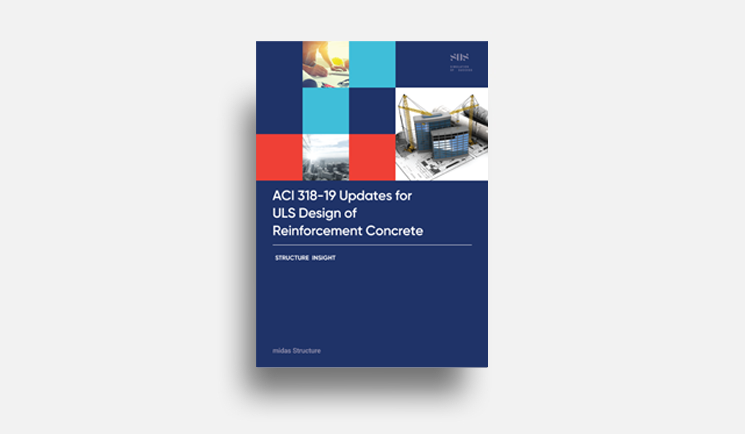# midas Structure

BLOG PROJECT TUTORIAL

# What is Element Rotation?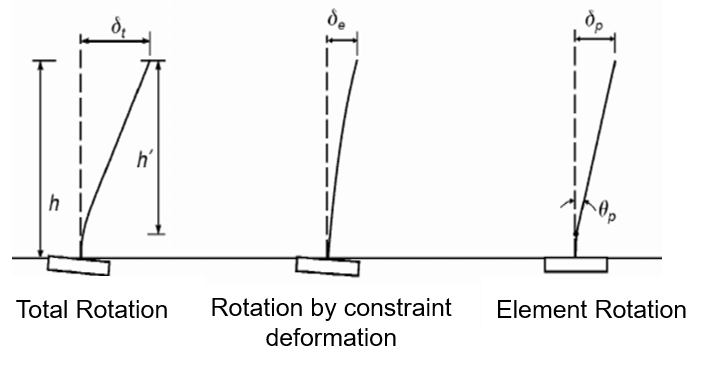Figure 1. Element Rotation Model

Element rotation means the angle caused according to the lateral deformation of the element.

However, the deformation by analysis includes the rotation value due to the deformation of the adjacent constraint as shown below.

Therefore, the element rotation can be calculated by removing the rotation by constrained deformation from the total rotation.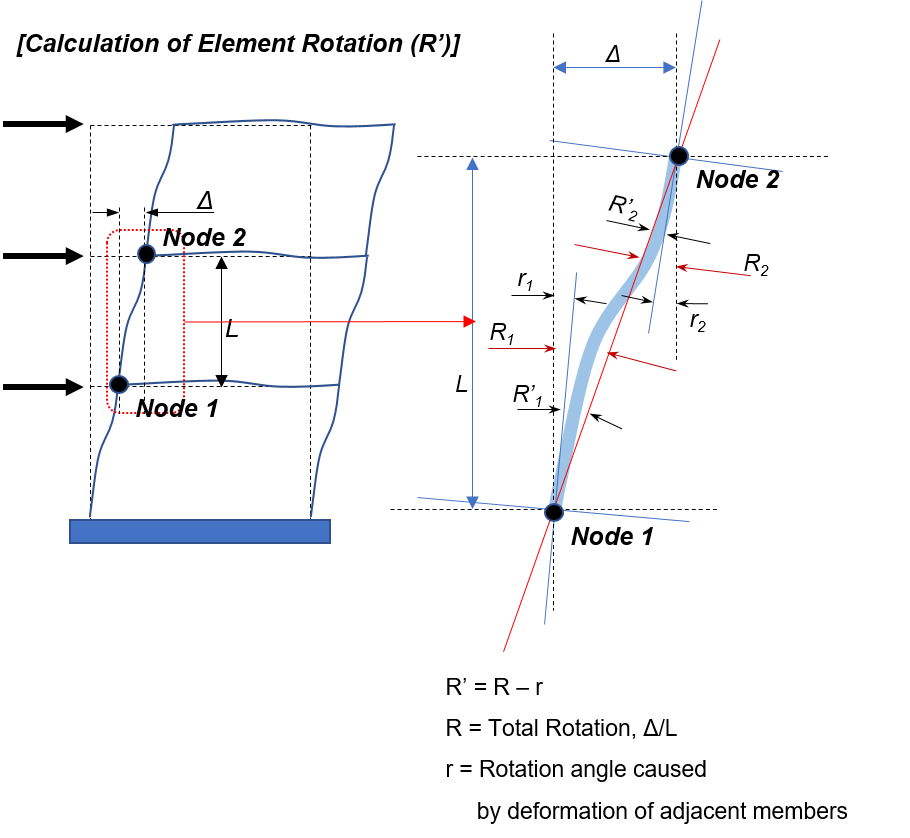Figure 2. Calculation of Element Rotation

# What is Hinge Rotation?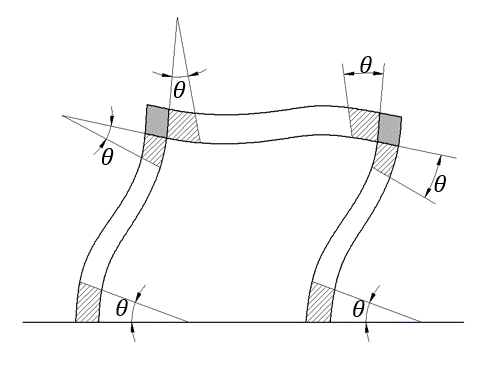Figure 3. Hinge Rotation Model

Hinge rotation means the curvature caused by the bending moment. It is usually considered where large moment occurs, and the hinges usually occur at the ends of the elements. Hinge rotation is calculated from the relationship between bending moment and stiffness of the element as shown on the right.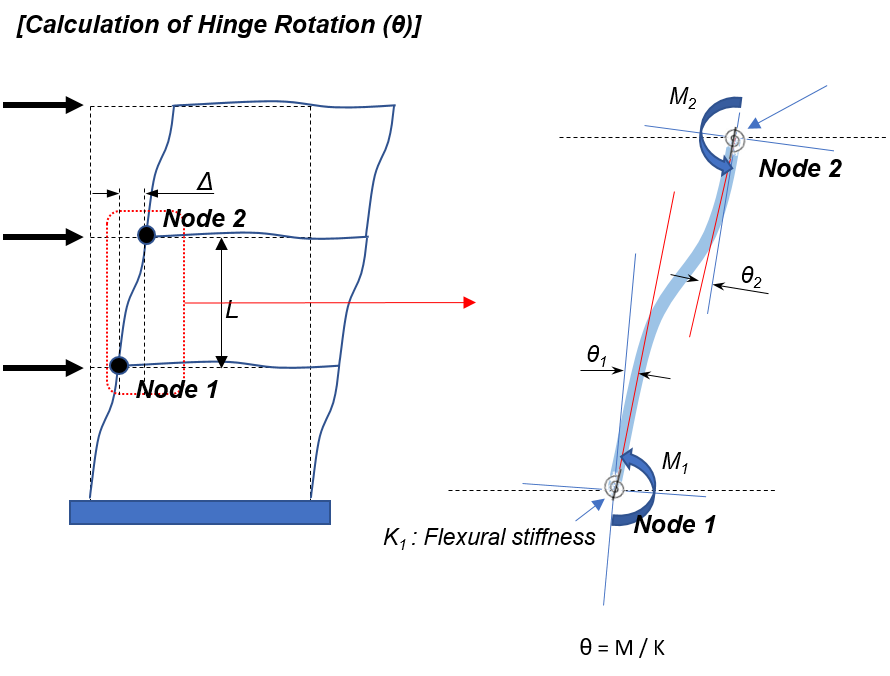Figure 4. Calculation of Hinge Rotation

# How to Calculate Element Rotation & Hinge Rotation in midas Gen

Example Model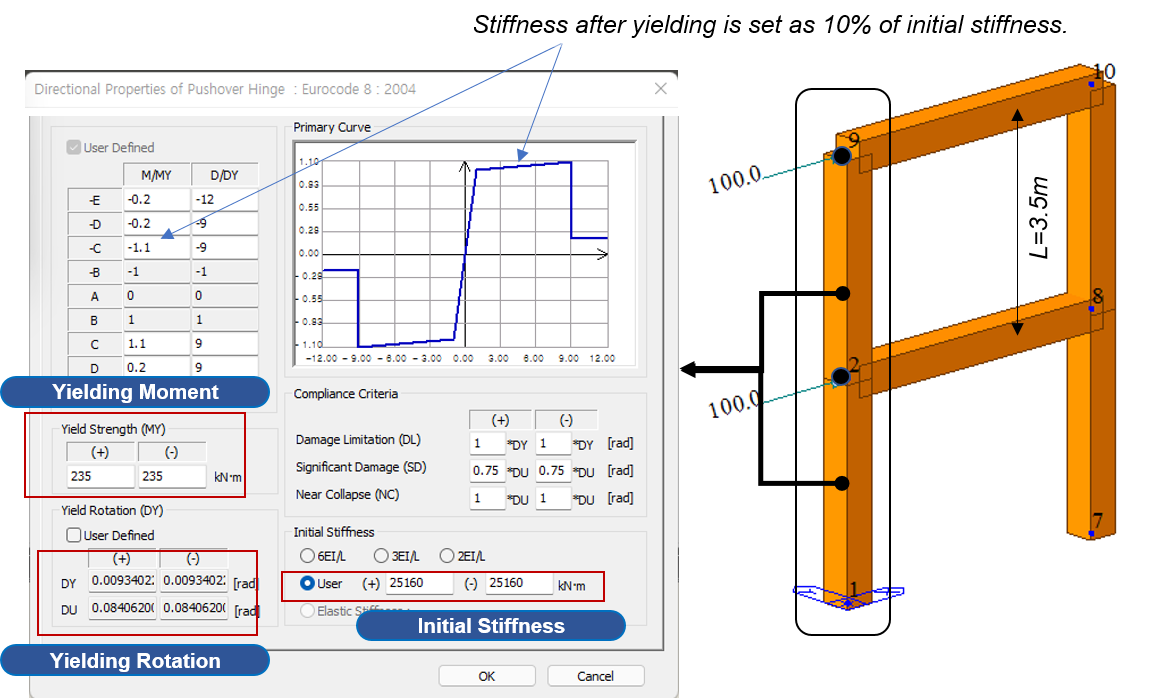Figure 5. Hinge Properties of Column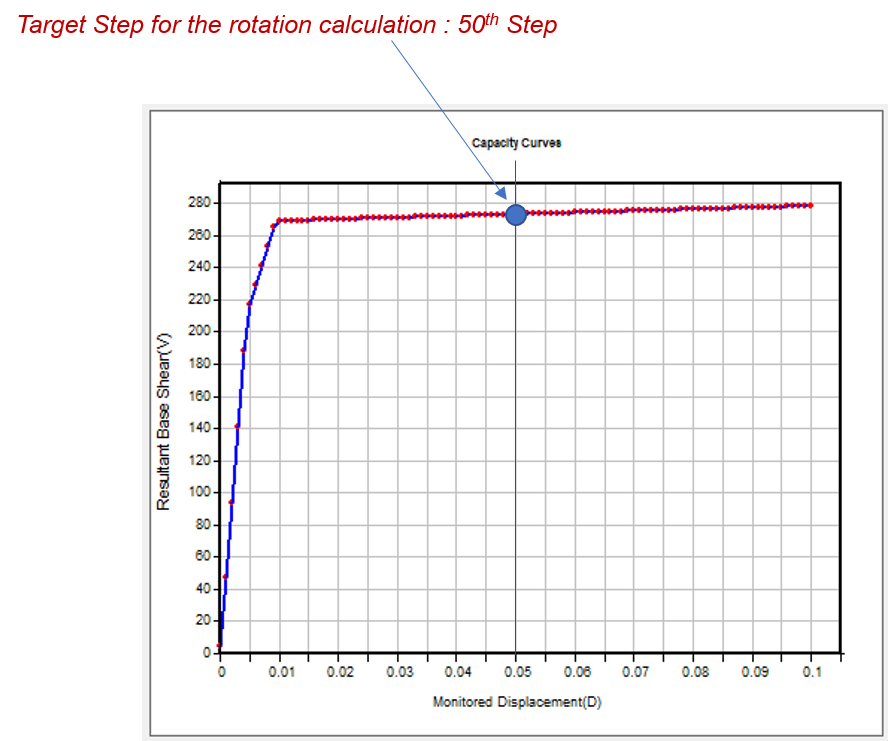Figure 6. Pushover Analysis Result

## How to Calculate Element Rotation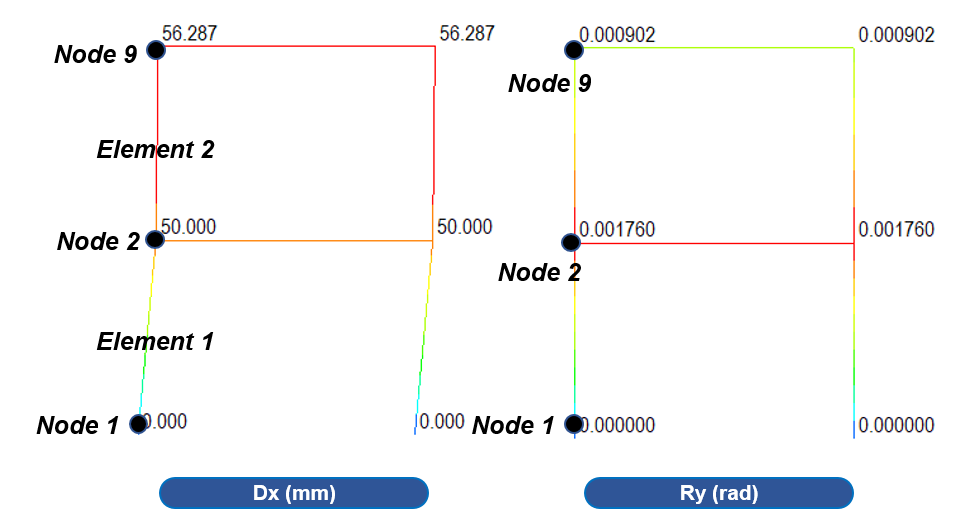Figure 7. Displacements at 50th Step

## Calculation of Element Rotation

** Note.
There is a slight difference in the midas Gen because the rotation by shear deformation is also removed.

 Location Lateral Displacement Rotation by Constraint Element No Node No Dx (mm) Ry (rad) 1 1 0.000 (Dxi) 0 2 50.000 (Dxj) 0.001760 2 2 50.000 (Dxi) 0.001760 9 56.287 (Dxj) 0.000902

 Location Element Rotation (rad) *(Dxi-Dxj)/Length - Ry Element No Node No 1 1 (50-0)/3500 – 0 = 0.0142857 2 (50-0)/3500 – 0.001760 = 0.0125257 2 2 (56-50)/3500 – 0.001760 = 0.0000363 9 (56-50)/3500 – 0.000902 = 0.0008943

## How to Calculate Hinge Rotation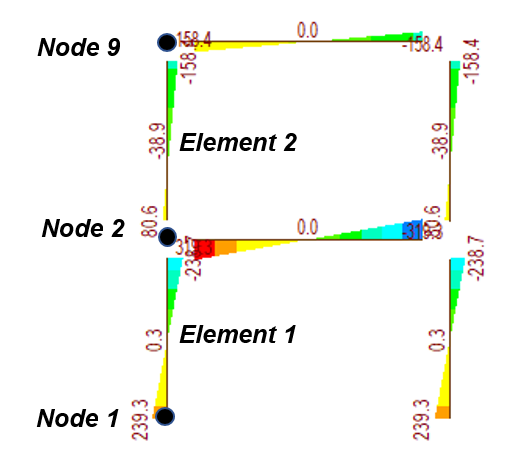Figure 8. Moment at 50th Step

 Location Moment at 50th step (Mu) Yielding Moment (My) Hinge Rotation (rad) Element No Node No Initial Stiffness (Ki) Stiffness after yielding (0.1*Ki) Elastic (θe) Plastic (θp) Total (θt = θe+θp) 1 1 239.3 235 25160 2516 0.009340 0.001709 0.011049 2 238.7 235 25160 2516 0.009340 0.001471 0.010811 2 2 50.6 235 25160 2516 0.002011 0 0.002011 9 158.4 235 25160 2516 0.006296 0 0.006296

• Elastic Hinge Rotation (θe) = Min(Mu, My) / Ki
• Plastic Hinge Rotation (θp) = Max(0, Mu – My) / 0.1*Ki
• Total Hinge Rotation = θe + θp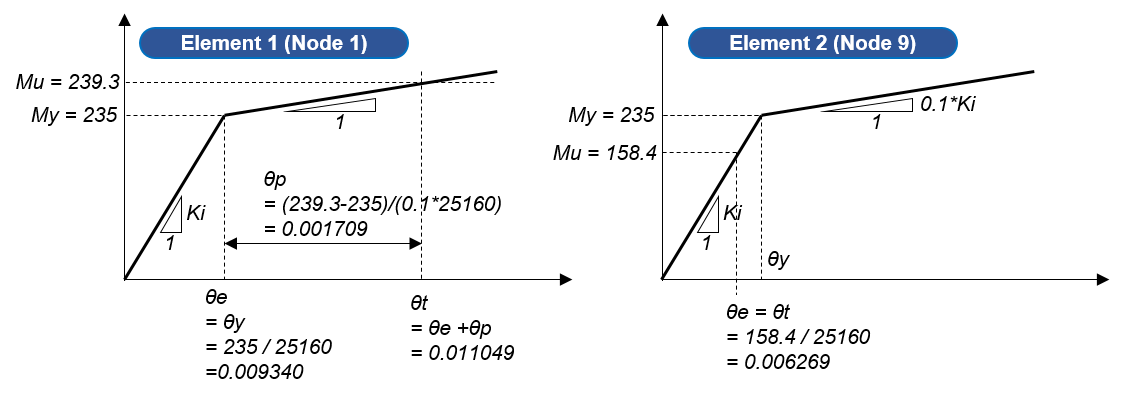Figure 9. Element 1 & 2 Model

# Where are Element Rotation and Hinge Rotation Used?

## Element Rotation - Eurocode 8-3 A.3.

Check 'Safety Verification' as per EC8-3 A.3.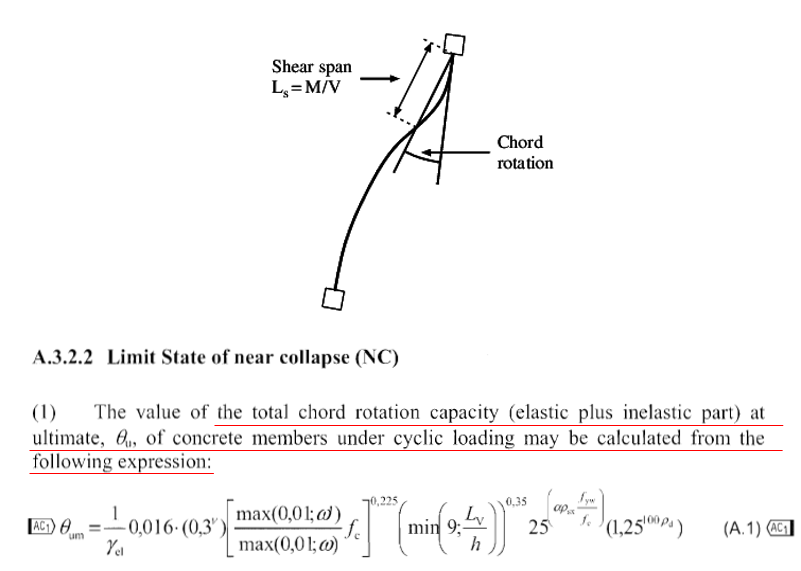Figure 10. Limit State of Near Collapse

* Element rotation (= chord rotation) < chord rotation Capacity (θum)

## Element Rotation - FEMA 356 C1.5.1

Check ‘Structural Performance Level and Damage’ as per EC8-3 A.3.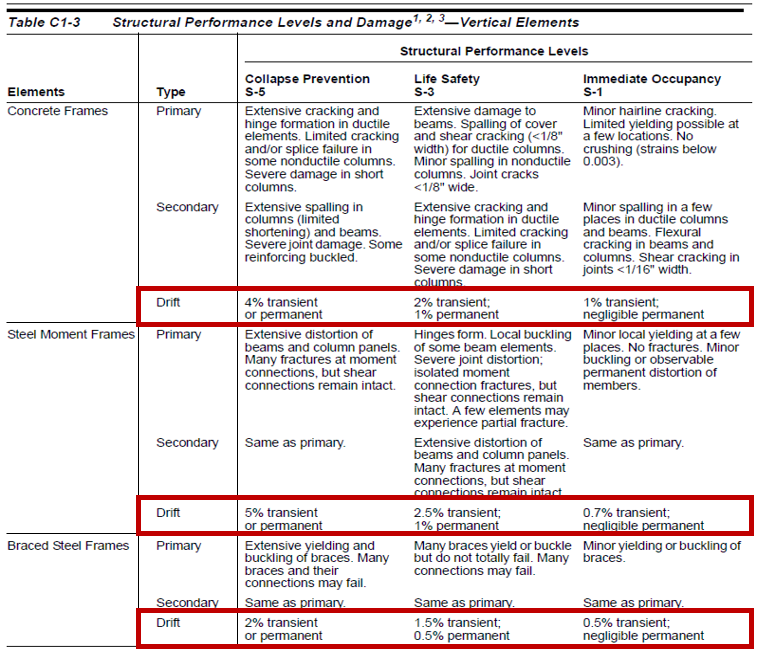Figure 11. Structural Performance Levels and Damage

## Hinge Rotation - FEMA 356 C1.5.1

Check ‘Analysis Result’ & ‘Acceptance Criteria’ as per FEMA 356.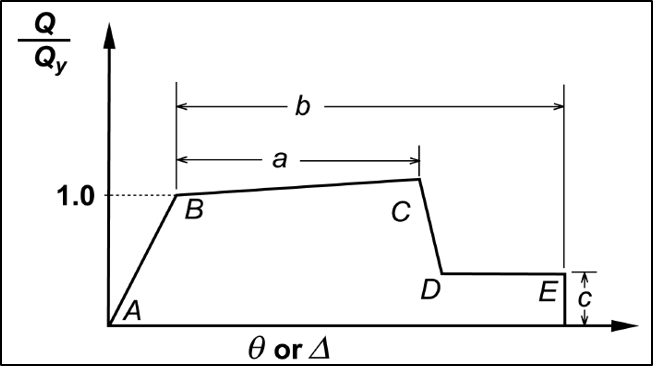Figure 12. Hinge Rotation Analysis Model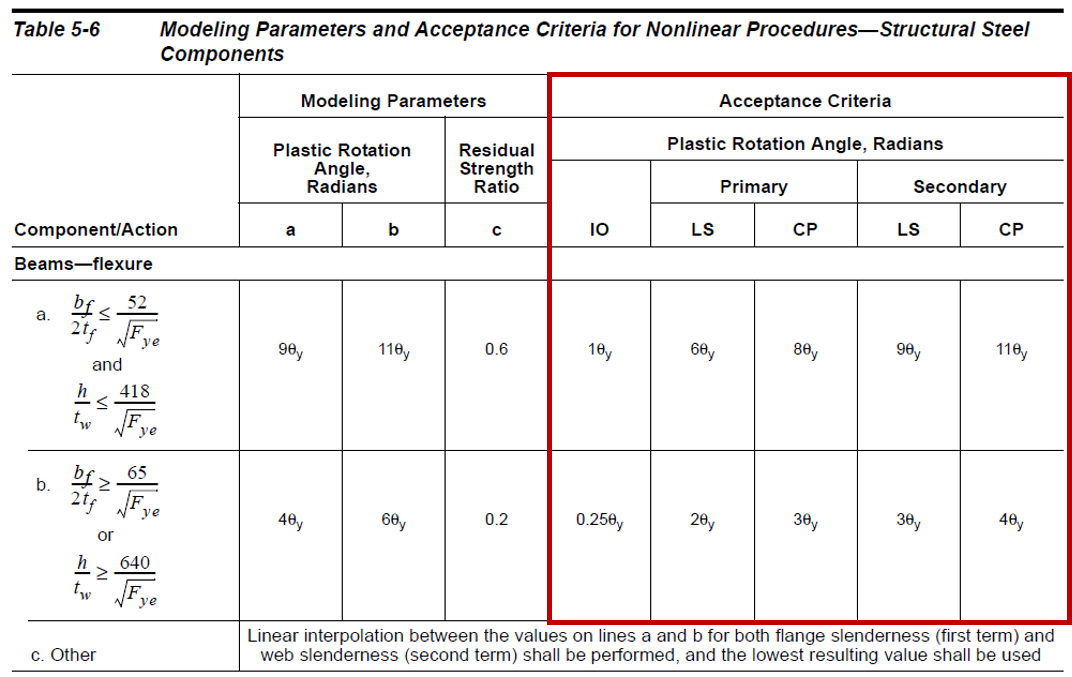Figure 13. Modeling Parameters and Acceptance Criteria for Nonlinear Procedures

Author InformationYeong-il Seo | Principal Structural Engineer

Young-il has over 13+ years of experience in building design, especially high-rise buildings with column reduction analysis, plant structures, pushover analysis, health monitoring, and vibration control projects. Since 2016, he is planning and providing technical supports for midas building products such as midas Gen, nGen, and Design+.